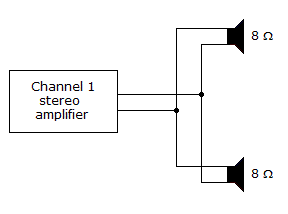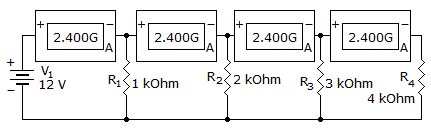# Electronics - Parallel Circuits

Exercise : Parallel Circuits - General Questions
6.
Components that connect in parallel form:
branches
open circuits
short circuits
a voltage divider
Explanation:
No answer description is available. Let's discuss.

7.
A parallel circuit differs from a series circuit in that a parallel circuit has
no path for current flow
fewer paths for current flow
one path for current flow
more than one path for current flow
Explanation:
No answer description is available. Let's discuss.

8.In the given circuit, Channel 1 of the stereo amplifier outputs 12 V to the speakers. How much total current is the amplifier providing to the speakers?

0 A
1.5 A
3 A
More information is needed to find the total current provided to the speakers.
Explanation:
No answer description is available. Let's discuss.

9.
If two parallel-connected resistors dissipate 6 watts and 10 watts of power, then what is the total power loss?
3.75 watts
4 watts
16 watts
60 watts
Explanation:
No answer description is available. Let's discuss.

10.
Which component is shorted?R1
R2
R3
R4